﻿ 关于非对称数据的ESMD改进算法 Improvement of ESMD Algorithm for Asymmetric Data

Vol. 07  No. 12 ( 2018 ), Article ID: 27923 , 6 pages
10.12677/AAM.2018.712174

Improvement of ESMD Algorithm for Asymmetric Data

Shuo Jiang, Jinliang Wang

Research Institute of ESMD Method and Its Application, School of Science, Qingdao University of Technology, Qingdao Shandong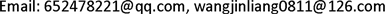Received: Nov. 12th, 2018; accepted: Dec. 3rd, 2018; published: Dec. 10th, 2018ABSTRACT

The ESMD method is the abbreviation of “Extreme-point Symmetric Mode Decomposition method”. It has its unique role in the research and engineering application of data processing involved in various research fields. But according to the principle of ESMD data analysis method, it is generally suitable for the more symmetrical data about the midpoint in every half period. For asymmetric (peak-valley asymmetry) data, the midpoint selected in this method is unrepresentative. In this paper, the ESMD method is improved for the analysis of this kind of data, and the local mean value per half period is used to replace the midpoint of maximum and minimum in the original algorithm, so that the asymmetric data can be better reflected. The numerical simulation proves the method’s feasibility and effectiveness.

Keywords:Extreme-Point Symmetric Mode Decomposition, Asymmetric Data, Hilbert-Huang Transformation Method, Data Analysis MethodsESMD方法是“极点对称模态分解方法”的简称，其在各个研究领域所涉及数据处理的科研和工程应用有其独特的作用。但是通过ESMD数据分析方法的原理可知，其一般适应于在每半个周期中关于中点较对称的数据。对于非对称(峰谷不对称)数据来说，该方法中选取的中点失去了代表性。本文针对这种数据的分析对ESMD方法进行了改进，用每半个周期的局部均值来代替原先算法中极大值与极小值的中点，这样可以更好的反应非对称数据的情况，并通过数值模拟验证了上述方法的可行性与有效性。1. 引言

ESMD   是英文“Extreme-point Symmetric Mode Decomposition”的简称，其中文意思是“极点对称模态分解”。该方法是希尔伯特-黄变换方法    的新发展，由两部分组成：第一部分是模态分解，可以产生数个模态与一条最佳的自适应全局均线；第二部分是时-频分析  。本文主要针对ESMD方法的第一部分进行改进，原方法具体计算步骤如下：

$\stackrel{¯}{Y}=\frac{1}{N}\underset{i=1}{\overset{N}{\sum }}{y}_{i}$ (1)

${\sigma }_{0}^{2}=\frac{1}{N}\underset{i=1}{\overset{N}{\sum }}{\left({y}_{i}-\stackrel{¯}{Y}\right)}^{2}$ . (2)

${\sigma }^{2}=\frac{1}{N}\underset{i=1}{\overset{N}{\sum }}{\left({y}_{i}-{r}_{i}\right)}^{2}$ (3)

$v=\frac{\sigma }{{\sigma }_{0}}$ (4)

ESMD方法适用于非线性、非平稳信号，如气候  、海气通量  等随机的时间序列数据，其在处理对称数据时取局部中点具有很好的代表性，分解之后的模态与自适应全局均线能准确的表达对称数据的信息与变化趋势。本文主要针对ESMD方法在处理非对称数据时所存在的缺陷对该方法进行改进。

2. ESMD方法在处理非对称数据时出现的问题及原因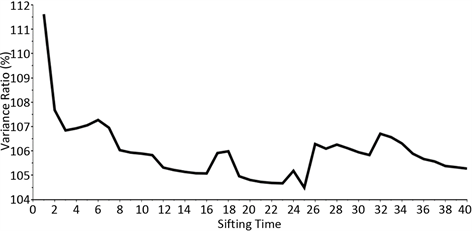Figure 1. Standard deviation ratio with different screening times (original method)

3. ESMD改进算法的实验结果与分析

${{\sigma }^{\prime }}^{2}=\frac{1}{N}\underset{i=1}{\overset{N}{\sum }}{\left({y}_{i}-{{r}^{\prime }}_{i}\right)}^{2}$ (5)

${v}^{\prime }={\sigma }^{\prime }/{\sigma }_{0}$ . (6)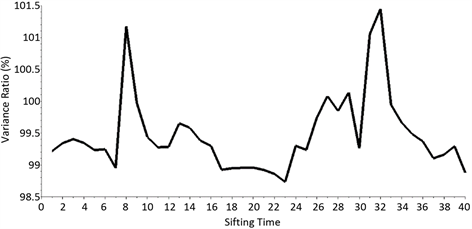Figure 2. Standard deviation ratio with different screening times (improved method)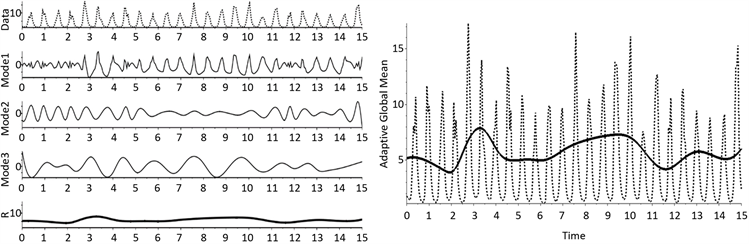(a) (b)

Figure 3. Modal decomposition and adaptive global mean (original method)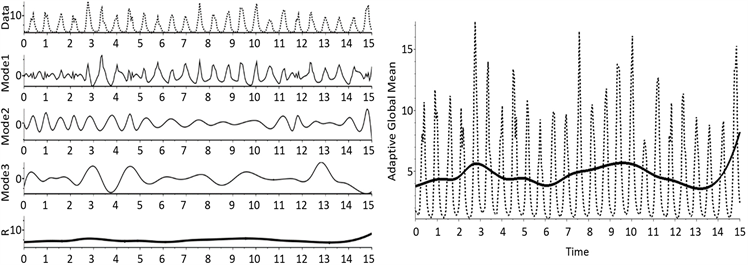(a) (b)

Figure 4. Modal decomposition and adaptive global averaging (improved method)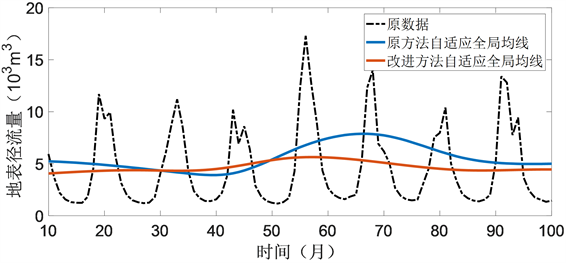Figure 5. Comparison between the original method and the improved method adaptive global moving averageTable 1. System resulting data of standard experiment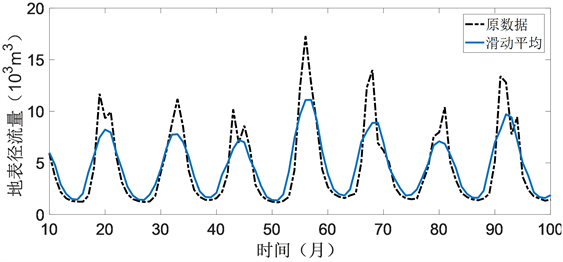Figure 6. 5 points sliding average processing was performed on the original data

4. 结论

Improvement of ESMD Algorithm for Asymmetric Data[J]. 应用数学进展, 2018, 07(12): 1500-1505. https://doi.org/10.12677/AAM.2018.712174

1. 1. Wang, J.L. and Li, Z.J. (2013) Extreme-Point Symmetric Mode Decomposition Method for Data Analysis. Advances in Adaptive Data Analysis, 5, 1350015. http://arxiv.org/abs/1303.6540

2. 2. 王金良, 李宗军. 极点对称模态分解方法: 数据分析与科学探索的新途径[M]. 北京: 高等教育出版社, 2015.

3. 3. Huang, N.E. and Shen, S.S.P. (1998) Hil-bert-Huang Transform: Introduction and Application. World Scientific, Singapore.

4. 4. Huang, N.E., Shen, Z., Long, S.R., et al. (1998) The Empirical Mode Decomposition and the Hilbert Spectrum for Nonlinear and Nonstationary Time Series Analysis. Proceedings of the Royal Society of London A, 454, 903-995. https://doi.org/10.1098/rspa.1998.0193

5. 5. Huang, N.E. and Wu, Z. (2005) A Review on Hilbert-Huang Trans-form: Method and Its Applications to Geophysical Studies. Reviews of Geophysics, 46, RG2006. https://doi.org/10.1029/2007RG000228

6. 6. 房贤水. 基于ESMD方法的模态统计特征研究[D]: [硕士学位论文]. 青岛: 青岛理工大学, 2015.

7. 7. Wang, J.L. and Li, Z.J. (2014) The ESMD Method for Climate Data Analysis. Climate Change Research Letters, 3, 1-5. https://doi.org/10.12677/CCRL.2014.31001

8. 8. Li, H.F., Wang, J.L. and Li, Z.J. (2013) Application of ESMD Method to Air-Sea Flux Investigation. International Journal of Geosciences, 4, 8-11. https://doi.org/10.4236/ijg.2013.45B002

9. 9. 茆诗松, 等. 概率论与数理统计教程[M]. 北京: 高等教育出版社, 2004.